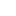### Uniform volume density between two planes - Corrected Exercises Gauss Theorem

Consider two infinite planes x = - a and x = a. The space between the two planes has a volume density of uniform and constant ρ loads. For x> a and x <- a, it reigns on vacuum .

1. Show that at any point of space, the electrostatic field of this distribution can be written.
2. Expressing Exfor the different parts of space and plot the Exa function of x.
3. Determine for each region the potential V (x) adopting V (0) = 0. Draw the graph of V (x) in terms of x.
4. It is assumed that a approaches 0and that the multiplication  ρaremains finite. Set an areal density limit load and look for Exon a classic result.

See solution